American Journal of Electromagnetics and Applications
Volume 4, Issue 1, September 2016, Pages: 8-13

Z Transform and FDTD Method in Unmagnetized Plasma

Khitam Y. Elwasife, Amal Y. Albatniji

Physics Department, Islamic University of Gaza, Gaza,Palestinian Authority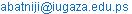(A. Y. Albatniji)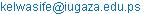(K. Y. Elwasife)

Khitam Y. Elwasife, Amal Y. Albatniji. Z Transform and FDTD Method in Unmagnetized Plasma. American Journal of Electromagnetics and Applications. Vol. 4, No. 1, 2016, pp. 8-13. doi: 10.11648/j.ajea.20160401.12

Received: October 7, 2016; Accepted: October 28, 2016; Published: November 16, 2016

Abstract: The Z transform is typically used in digital filtering and signal processing problems. The paper illustrates the use of the Z transform in implementing the FDTD method where it is useful to avoid dealing with convolution integral in the time domain by going immediately to Z domain. This work study the pulse propagation in free space that comes upon a plasma. Plasma is very interesting medium and the results illustrated that at low frequencies, it looks like a metal, and higher frequency, it become transparent just like a dielectric medium.

Keywords: Z Transform, Finite Difference Time Domain, Unmagnified Plasma

Contents

1. Introduction

The finite-difference time-domain (FDTD) solution procedure developed by Yee , Recently has in been extended to dispersive and anisotropic media to handle materials such as magnetized ferrites and plasmas. The solution for dispersive media has been accomplished through a recursive update of a convolution integral in the constitutive relations for the fields of FDTD simulation , , ,  by numerical solution of the differential equation form of the constitutive relations  and  and using Z transforms . The basic techniques and important concepts of the one-dimensional electromagnetic particle are studied . Maxwell’s equations and equations of motions for a large number of super particles are solved. Electromagnetic propagation through a magnetized plasma is studied with the finite-difference time-domain (FDTD) method by means of Z transforms. The propagation direction is perpendicular to the biasing magnetic filed and the electric field is parallel to the biasing magnetic filed. The dependence of the reflection and transmission coefficients on frequency is obtained by discrete Fourier transform. A comparison with analytical values of homogeneous magnetized plasma is made. The reflection and transmission coefficients in an inhomogeneous magnetized plasma layer are calculated . A novel and normalized Z-transform-based finite-difference time-domain (ZTFDTD) method is presented for simulating the interaction of the electromagnetic (EM) wave with unmagnetized plasma . The 2-D ZTFDTD formulations for unmagnetized plasma are derived. Using a simplified 2-D model for a perfectly conducting cylinder covered with unmagnetized plasma, the stealth effect of unmagnetized plasma is studied in different thicknesses of plasma, electron densities of plasma, EM wave frequencies, and plasma collision frequencies. Numerical results indicate that plasma stealth is effective in theory and reasonable selection for the plasma parameters can greatly enhance its effectiveness.

2. Theory

The dielectric constant and conductivity of most media vary at different frequencies. The model consider is a plasma medium cover with free space in both side as shown in Fig. 1.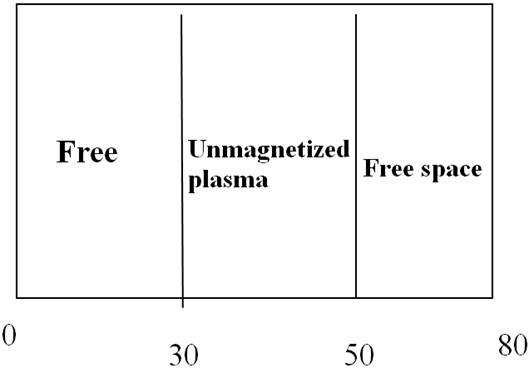Figure 1. Unmagnetized Plasma sandwich between two free space layer, it represent the region from 300-500 position in X-axis.

The pulse we have using is the Gaussian pulse which contain spectrum of frequencies. One of the most significant development in the FDTD method by means to simulate frequency dependent material. We simulate the of electromagnetic wave in unmagnified plasma, the permittivity of the unmagnetized plasma is given as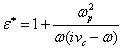(1)

Where:

ωp =2πfp

fp is the plasma frequency

vc is the electron collision frequency.

Using partial fraction expansion Equation 1, becomes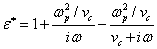(2)

And lets start by taking Z transform of equation (2) to obtain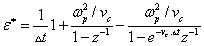(3)

We use Maxwell's equation in the form of flux density as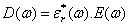(4)

Wher E is the electric field. By the convolution theorem the Z -transform of equation (4) is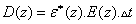(5)

Inserting equation (3) in equation (4) we obtain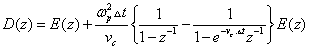(6)(7)

E(z), the electric field as a function of Z can be solved for by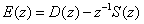(8)

Where S(z) can written as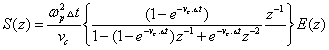(9)

Therefore, the FDTD Simulation becomes: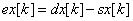(10)

3. Results and Discussion

The finite-different time-domain method has been widely used to simulate the transient solutions of electromagnetic wave propagation in many media according to its constant properties. Here we have assumed one dimensional unmagnetized plasma with permittivity dependent frequency. In time-domain, the electromagnetic propagation process of a Gaussian pulse through an unmagnetized plasma We have been simulated the Plasma medium by sinusoidal pulse with different frequencies such as 500π THz, 2000500π THz, 4000500π THz, and 5000500π THz. Absorbing material boundary condition is used in these simulations. vc is the electron collision frequency. The electric field of different time steps has been plotted. The low or high frequencies which we use is equal 2000 THz and Vc=57 THz. It means below or above the Plasma frequency. Fig. 1 Simulation of electromagnetic waves propagation in free space and striking a plasma medium. The cell size is dx=75µm and vc is 0.2 THz. The propagation wave has a center frequency of 500πGHz. After 650 time steps, it is starts to strike plasma layer and start to reflect, but at 750 steps it is reflected as in Fig. 2. and in Fig. 3. it completely reflected when it reach 850 time steps. Fig. 4 Illustrates simulation of wave propagation in free space and stricking a plasma medium. The cell size is dx=1nm and vc is 0.2 THz. The propagation wave has a center frequency of 4000πTHz. After 800 time steps, it is almost inter plasma medium. In Fig. 5. and Fig. 6. we illustrated the pulse with frequency of 4000πTHz. And 5000πTHz respectively After 1300 time steps, it has completely passed plasma medium but each signal is different in their amplitude the transmission for pulse of sinusoidal electromagnetic waves shown in Fig. 6 and Fig. 7 after 1300 and 1200 time steps respectively. In Fig. 8 The propagation wave has a center frequency of 2000πTHz. After 565 time steps, it start to strike plasma medium. Also The propagation wave has a center frequency of 2000πTHz. After 900 time steps, it has passed completely the plasma medium without any reflected as shown in Fig. 9. We show that in Fig. 10 and 11, the propagation wave has a center frequency of 2000πTHz. After 1100 and 1300 time steps respectively, it has passed completely the plasma medium without any reflected.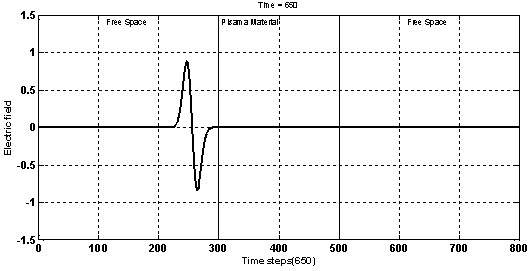Figure 2. Simulation of wave propagation in free space and striking a plasma medium. The cell size is dx=75nm and vc is 0.2 THz. The propagation wave has a center frequency of 500πGHz. After 650 time steps, it is almost completely reflected.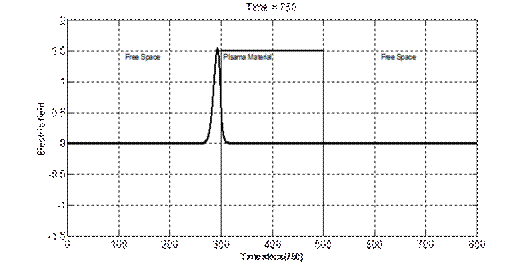Figure 3. Simulation of wave propagation in free space and striking a plasma medium. The cell size is dx=75nm and vc is 0.2 THz. a center frequency of 500πGHz. After 750 time steps, it is almost completely reflected.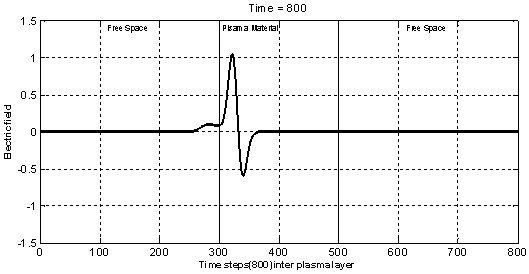Figure 4. Simulation of wave propagation in free space and striking a plasma medium. The cell size is dx=1nm and vc is 0.2 THz. The propagation wave has a center frequency of 4000πTHz. After 800 time steps, it is completely inter plasma medium.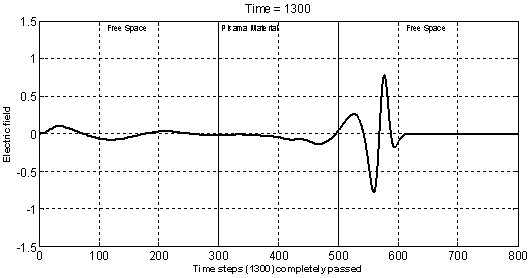Figure 5. Simulation of wave propagation in free space and striking a plasma medium. The cell size is dx=1nm and vc is 0.2 THz. The propagation wave has a center frequency of 4000πTHz. After 1300 time steps, it has completely passed plasma medium.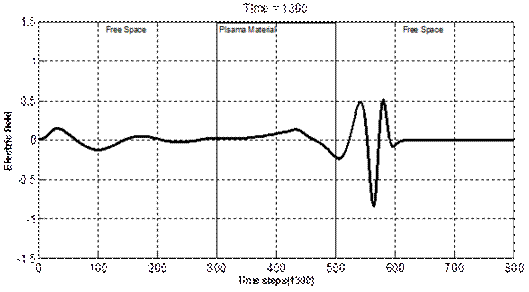Figure 6. Simulation of wave propagation in free space and striking a plasma medium. The cell size is dx=1nm and vc is 0.2 THz. The propagation wave has a center frequency of 5000πTHz. After 1300 time steps, it has passed completely plasma medium.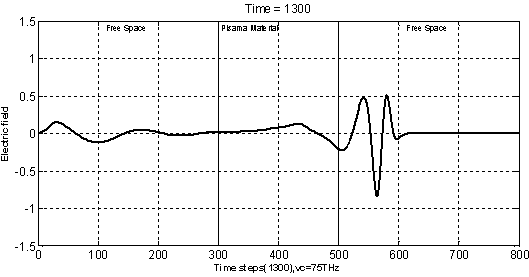Figure 7. Simulation of wave propagation in free space and striking a plasma medium. The cell size is dx=1nm and vs. is 75 THz. The propagation wave has a center frequency of 5000πTHz. After 1200 time steps, it has passed completely plasma medium.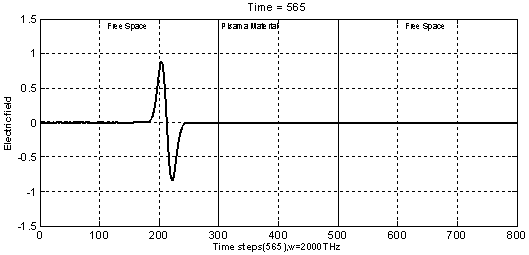Figure 8. Simulation of wave propagation in free space and striking a plasma medium. The cell size is dx=1nm and vc is 75 THz. The propagation wave has a center frequency of 2000πTHz. After 565 time steps, it starts to strike plasma medium.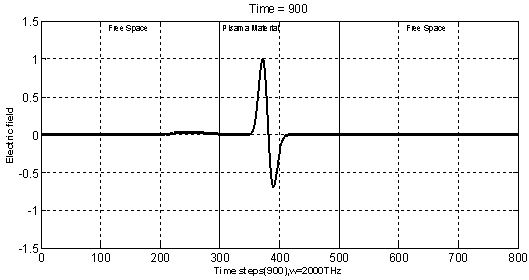Figure 9. Simulation of wave propagation in free space and striking a plasma medium. The cell size is dx=1nm and vc is 75 THz. The propagation wave has a center frequency of 2000πTHz. After 900 time steps, it has passed completely plasma medium without any reflected.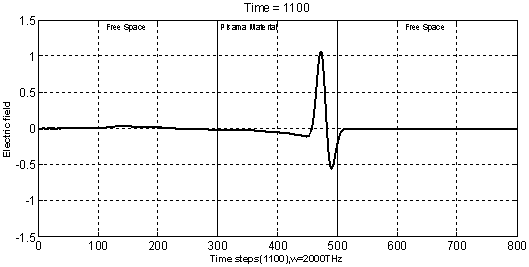Figure 10. Simulation of wave propagation in free space and striking a plasma medium. The cell size is dx=1nm and vc is 75 THz. The propagation wave has a center frequency of 2000πTHz. After 1100 time steps, it has passed completely plasma medium without any reflection.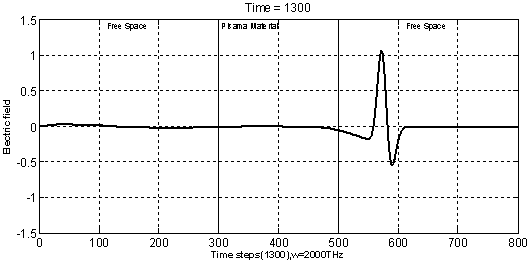Figurer 11. Simulation of wave propagation in free space and striking a plasma medium. The cell size is dx=1nm and vc is 75 THz. The propagation wave has a center frequency of 2000πTHz. After 1300 time steps, it has passed completely plasma medium without any reflection.

4. Conclusion

In this work we use sinusoidal pulse to simulate and study the behavior of electromagnetic waves sticking unmagnetized plasma medium for different frequencies and many time steps. We study the electric field through a plasma medium which a magnetic field is not strong enough to influence the motion of the charged particles. At low frequency the medium looks like a metal and the medium becomes transparent just like a dielectric medium at high frequency. The frequency which is high or low with respect to plasma frequency.

References

1. K. S. Yee, "Numerical solution of initial boundary value problems involving Maxwell’s equations in isotropic media", IBEE. Antennas Propagate., Vol. AP-14, 1966,pp. 302-307.
2. R. J. Luebbers, F. P. Hunsberger, K. S. Kunz, R. B. Standler and M. Schneider, "A fiequencydependent finite-difference time-domain formulation for dispersive materials", hms.Electromagn. Compat., Vol. EMC-32, 1990, pp. 222-227.
3. R. J. Luebbers and F. P. Hupsberger, "FDTD for Nth-Order Dispersive Media", IEEE. Antennas Propagat., Vol. AP-40,1992, pp. 1297-1301.
4. K. S. Kunz and R. J. Luebbers, "The Finite Diflemnce Time Domain Method for E2ectromagnetics", CRC Press, Boca Raton, 1993.
5. R. Luebbers, D. Steich and K. Kunz, "FDTD calculation of scattering from frequencydependent materials", IEEE I%zns.Antennas.,Vol. AP-41,1993, pp. 1249-1257.
6. T. Kashiwa and I. FuM, "A treatment of the dispersive characteristics associated with electronic polarization", Microwave Opt. Techno2.Lett., Vol. 3, No. 6, 1990, p. 203.
7. R. Joseph, S. Hagness and A. Taflove, "Direct time integration of Maxwell’s equations in linear dispersive media with absorption for sattering ad propagation of ferntosecond-tromagnetic pulses",  Opt. Lett., Vol. 16, no. 18, 1991, p. 1412.
8. D. M. Sullivan, "A Frequency-Dependent FDTD Method for Biological Applications", IEEE tins. Microwave Thw~ Tech., Vol. 40, 1992, pp. 532-539.
9. Yoshiharu Omura,"One-dimensional Electromagnetic Particle Code: KEMPO", Advanced Methods for Space Simulations,2007, pp. 1–21.
10. Huang Shou-jiang, "A Finite-Difference Time-Domain Analysis of Electromagnetic Propagation in Magnetized Plasmas",Chinese Journal of Computation Physics.ISSN:1001-2005, p.246.
11. Ming Yan,"Z-Transform-Based FDTD Analysis of Perfectly Conducting Cylinder Covered With Unmagnetized Plasma", Journals of Magnetics, IEEE Transactions. Vol. 43, Issue:6,2007, P.2968 -2970.

 Contents 1. 2. 3. 4.
Article ToolsAbstractPDF(288K)